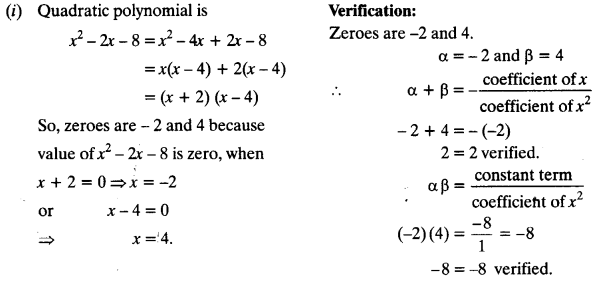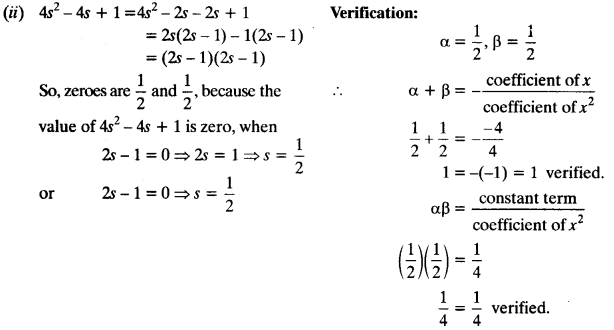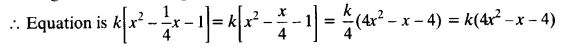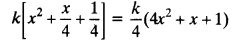## NCERT Solutions for Class 10 Maths Chapter 2 Polynomials Ex 2.2

NCERT Solutions for Class 10 Maths Chapter 2 Polynomials Ex 2.2 are part of NCERT Solutions for Class 10 Maths. Here we have given NCERT Solutions for Class 10 Maths Chapter 2 Polynomials Ex 2.2.

 Board CBSE Textbook NCERT Class Class 10 Subject Maths Chapter Chapter 2 Chapter Name Polynomials Exercise Ex 2.2 Number of Questions Solved 2 Category NCERT Solutions

### NCERT Solutions for Class 10 Maths Chapter 2 Polynomials Ex 2.2

Question 1.
Find the zeroes of the following quadratic polynomials and verify the relationship between the zeroes and the coefficients.
(i) x2 – 2x – 8
(ii) 4s2 – 4s + 1
(iii) 6x2 – 3 – 7x
(iv) 4u2 + 8u
(v) t2 -15
(vi) 3x2 – x – 4
Solution:Question 2.
Find a quadratic polynomial each with the given numbers as the sum and product of its zeroes respectively.Solution:
(i) Zeroes of polynomial are not given, sum of zeroes = $$\frac { 1 }{ 4 }$$ and product of zeroes = -1
If ax2 + bx + c is a quadratic polynomial, then
α + β = sum of zeroes = $$\frac { -b }{ a }$$ = $$\frac { 1 }{ 4 }$$ and αβ = product of zeroes = $$\frac { c }{ a }$$ = -1
Quadratic polynomial is ax2 + bx + c
Let a = k, ∴ b = $$\frac { -k }{ 4 }$$ and c = -k
Putting these values, we getFor different values of k, we can have quadratic polynomials all having sum of zeroes as $$\frac { 1 }{ 4 }$$ and product of zeroes as -1.

(ii) Sum of zeroes = α + β = √2 = $$\frac { -b }{ a }$$; product of zeroes = αβ = $$\frac { 1 }{ 3 }$$ = $$\frac { c }{ a }$$
Quadratic polynomial is ax2 + bx + c
Let a = k,b = -√2k and c = $$\frac { k }{ 3 }$$
Putting these values we getFor all different real values of k, we can have different quadratic polynomials of the form 3×2 – 3√2x +1 having sum of zeroes = √2 and product of zeroes = $$\frac { 1 }{ 3 }$$

(iii) Sum of zeroes = α + β = 0 = $$\frac { -b }{ a }$$; product of zeroes = αβ = √5 = $$\frac { c }{ a }$$
Let quadratic polynomial is ax2 + bx + c
Let a = k,b = 0, c = √5 k
Putting these values, we get
k[x2 – 0x + √5 ] = k(x2 + √5).
For different real values of k, we can have different quadratic polynomials of the form
x2 + √5, having sum of zeroes = 0 and product of zeroes = √5

(iv) Sum of zeroes = α + β = 1= $$\frac { -b }{ a }$$; product of zeroes = αβ = 1 = $$\frac { c }{ a }$$
Let quadratic polynomial is ax2 + bx + c.
Let a=k, c = k, b = -k
Putting these values, we get k[x2 -x +1]
Quadratic polynomial is of the form x2 -x + 1 for different values of k.

(v) Sum of zeroes = α + β = $$\frac { -1 }{ 4 }$$= $$\frac { -b }{ a }$$; product of zeroes = αβ = $$\frac { 1 }{ 4 }$$ = $$\frac { c }{ a }$$
Let quadratic polynomial is ax2 + bx + c
Let a=k, b= $$\frac { k }{ 4 }$$, c= $$\frac { k }{ 4 }$$
Putting these values, we get kQuadratic polynomial is of the form 4x2 +x + 1 for different values of k.

(vi) Sum of zeroes = α + β = 4 = $$\frac { -b }{ a }$$; product of zeroes = αβ = 1 = $$\frac { c }{ a }$$
Let quadratic polynomial is ax2 + bx + c
Let a = k,b = -4k and c = k
Putting these values, we get
k[x2 – 4x + 1]
Quadratic polynomial is of the form x2 – 4x + 1 for different values of k.

We hope the NCERT Solutions for Class 10 Maths Chapter 2 Polynomials Ex 2.2 help you. If you have any query regarding NCERT Solutions for Class 10 Maths Chapter 2 Polynomials Ex 2.2, drop a comment below and we will get back to you at the earliest.